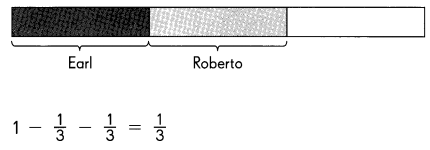# Math in Focus Grade 5 Chapter 4 Answer Key Multiplying and Dividing Fractions and Mixed Numbers

Go through the Math in Focus Grade 5 Workbook Answer Key Chapter 4 Multiplying and Dividing Fractions and Mixed Numbers to finish your assignments.

## Math in Focus Grade 5 Chapter 4 Answer Key Multiplying and Dividing Fractions and Mixed Numbers

Math Journal

Rachel drew a model to solve this problem:

Earl pours $$\frac{1}{3}$$ of a bottle of juice into his glass. Roberto pours $$\frac{1}{3}$$ of the remainder into his glass. What fraction of the bottle of juice is left?$$\frac{1}{3}$$ of the bottle of juice is left.
Did Rachel solve the problem correctly? Explain.
Yes. Rachel solve the problem correctly
Explanation:
$$\frac{1}{3}$$ + $$\frac{1}{3}$$  = $$\frac{2}{3}$$
1 – $$\frac{2}{3}$$ = $$\frac{1}{3}$$

Challenging Practice

An art teacher has a box of markers. She keeps half of the markers in the box and gives $$\frac{1}{3}$$ of the other half to group A. The remaining markers were shared equally among the 8 students in group B. What fraction of the whole box does each of the students in group B get?
She keeps$$\frac{1}{2}$$ $$\frac{3}{6}$$ in the box, gives $$\frac{1}{3}$$ of half ($$\frac{1}{6}$$ of box) to
Group A., the remaining $$\frac{2}{3}$$ of a half box ($$\frac{2}{6}$$ of box) to Group B.
Each student gets $$\frac{1}{8}$$ of $$\frac{2}{6}$$ of the box:
$$\frac{1}{8}$$ x $$\frac{2}{6}$$ = $$\frac{2}{48}$$ = $$\frac{1}{24}$$
Each Group B student gets $$\frac{1}{24}$$ of the box.
Mimi’s Market sold 24 heads of lettuce one morning. That afternoon $$\frac{2}{7}$$ of the remaining heads of lettuce were sold. The number of heads left was now $$\frac{1}{2}$$ of the number the market had at the beginning of the day. How many heads of lettuce were there at the beginning of the day?